$\newcommand{\NN}{\mathbb{N}} \newcommand{\CC}{\mathbb{C}} \newcommand{\GG}{\mathbb{G}} \newcommand{\LL}{\mathbb{L}} \newcommand{\PP}{\mathbb{P}} \newcommand{\QQ}{\mathbb{Q}} \newcommand{\RR}{\mathbb{R}} \newcommand{\VV}{\mathbb{V}} \newcommand{\ZZ}{\mathbb{Z}} \newcommand{\FF}{\mathbb{F}} \newcommand{\KK}{\mathbb{K}} \newcommand{\UU}{\mathbb{U}} \newcommand{\EE}{\mathbb{E}} \newcommand{\Aa}{\mathcal{A}} \newcommand{\Bb}{\mathcal{B}} \newcommand{\Cc}{\mathcal{C}} \newcommand{\Dd}{\mathcal{D}} \newcommand{\Ee}{\mathcal{E}} \newcommand{\Ff}{\mathcal{F}} \newcommand{\Gg}{\mathcal{G}} \newcommand{\Hh}{\mathcal{H}} \newcommand{\Ii}{\mathcal{I}} \newcommand{\Jj}{\mathcal{J}} \newcommand{\Kk}{\mathcal{K}} \newcommand{\Ll}{\mathcal{L}} \newcommand{\Mm}{\mathcal{M}} \newcommand{\Nn}{\mathcal{N}} \newcommand{\Oo}{\mathcal{O}} \newcommand{\Pp}{\mathcal{P}} \newcommand{\Qq}{\mathcal{Q}} \newcommand{\Rr}{\mathcal{R}} \newcommand{\Ss}{\mathcal{S}} \newcommand{\Tt}{\mathcal{T}} \newcommand{\Uu}{\mathcal{U}} \newcommand{\Vv}{\mathcal{V}} \newcommand{\Ww}{\mathcal{W}} \newcommand{\Xx}{\mathcal{X}} \newcommand{\Yy}{\mathcal{Y}} \newcommand{\Zz}{\mathcal{Z}} \newcommand{\al}{\alpha} \newcommand{\la}{\lambda} \newcommand{\ga}{\gamma} \newcommand{\Ga}{\Gamma} \newcommand{\La}{\Lambda} \newcommand{\Si}{\Sigma} \newcommand{\si}{\sigma} \newcommand{\be}{\beta} \newcommand{\de}{\delta} \newcommand{\De}{\Delta} \renewcommand{\phi}{\varphi} \renewcommand{\th}{\theta} \newcommand{\om}{\omega} \newcommand{\Om}{\Omega} \renewcommand{\epsilon}{\varepsilon} \newcommand{\Calpha}{\mathrm{C}^\al} \newcommand{\Cbeta}{\mathrm{C}^\be} \newcommand{\Cal}{\text{C}^\al} \newcommand{\Cdeux}{\text{C}^{2}} \newcommand{\Cun}{\text{C}^{1}} \newcommand{\Calt}{\text{C}^{#1}} \newcommand{\lun}{\ell^1} \newcommand{\ldeux}{\ell^2} \newcommand{\linf}{\ell^\infty} \newcommand{\ldeuxj}{{\ldeux_j}} \newcommand{\Lun}{\text{\upshape L}^1} \newcommand{\Ldeux}{\text{\upshape L}^2} \newcommand{\Lp}{\text{\upshape L}^p} \newcommand{\Lq}{\text{\upshape L}^q} \newcommand{\Linf}{\text{\upshape L}^\infty} \newcommand{\lzero}{\ell^0} \newcommand{\lp}{\ell^p} \renewcommand{\d}{\ins{d}} \newcommand{\Grad}{\text{Grad}} \newcommand{\grad}{\text{grad}} \renewcommand{\div}{\text{div}} \newcommand{\diag}{\text{diag}} \newcommand{\pd}{ \frac{ \partial #1}{\partial #2} } \newcommand{\pdd}{ \frac{ \partial^2 #1}{\partial #2^2} } \newcommand{\dotp}{\langle #1,\,#2\rangle} \newcommand{\norm}{|\!| #1 |\!|} \newcommand{\normi}{\norm{#1}_{\infty}} \newcommand{\normu}{\norm{#1}_{1}} \newcommand{\normz}{\norm{#1}_{0}} \newcommand{\abs}{\vert #1 \vert} \newcommand{\argmin}{\text{argmin}} \newcommand{\argmax}{\text{argmax}} \newcommand{\uargmin}{\underset{#1}{\argmin}\;} \newcommand{\uargmax}{\underset{#1}{\argmax}\;} \newcommand{\umin}{\underset{#1}{\min}\;} \newcommand{\umax}{\underset{#1}{\max}\;} \newcommand{\pa}{\left( #1 \right)} \newcommand{\choice}{ \left\{ \begin{array}{l} #1 \end{array} \right. } \newcommand{\enscond}{ \left\{ #1 \;:\; #2 \right\} } \newcommand{\qandq}{ \quad \text{and} \quad } \newcommand{\qqandqq}{ \qquad \text{and} \qquad } \newcommand{\qifq}{ \quad \text{if} \quad } \newcommand{\qqifqq}{ \qquad \text{if} \qquad } \newcommand{\qwhereq}{ \quad \text{where} \quad } \newcommand{\qqwhereqq}{ \qquad \text{where} \qquad } \newcommand{\qwithq}{ \quad \text{with} \quad } \newcommand{\qqwithqq}{ \qquad \text{with} \qquad } \newcommand{\qforq}{ \quad \text{for} \quad } \newcommand{\qqforqq}{ \qquad \text{for} \qquad } \newcommand{\qqsinceqq}{ \qquad \text{since} \qquad } \newcommand{\qsinceq}{ \quad \text{since} \quad } \newcommand{\qarrq}{\quad\Longrightarrow\quad} \newcommand{\qqarrqq}{\quad\Longrightarrow\quad} \newcommand{\qiffq}{\quad\Longleftrightarrow\quad} \newcommand{\qqiffqq}{\qquad\Longleftrightarrow\qquad} \newcommand{\qsubjq}{ \quad \text{subject to} \quad } \newcommand{\qqsubjqq}{ \qquad \text{subject to} \qquad }$

Signal and Image Noise Models

# Signal and Image Noise Models

This numerical tour show several models for signal and image noise. It shows how to estimate the noise level for a Gaussian additive noise on a natural image. It also shows the relevance of thresholding to remove Gaussian noise contaminating sparse data.

## Installing toolboxes and setting up the path.

You need to download the following files: signal toolbox and general toolbox.

You need to unzip these toolboxes in your working directory, so that you have toolbox_signal and toolbox_general in your directory.

For Scilab user: you must replace the Matlab comment '%' by its Scilab counterpart '//'.

Recommandation: You should create a text file named for instance numericaltour.sce (in Scilab) or numericaltour.m (in Matlab) to write all the Scilab/Matlab command you want to execute. Then, simply run exec('numericaltour.sce'); (in Scilab) or numericaltour; (in Matlab) to run the commands.

Execute this line only if you are using Matlab.

getd = @(p)path(p,path); % scilab users must *not* execute this


Then you can add the toolboxes to the path.

getd('toolbox_signal/');
getd('toolbox_general/');


The simplest noise model consist in adding a realization of a zero mean random vector to a clean signal or image.

N = 128;
name = 'boat';
M0 = rescale(crop(M0,N));


n = 1024;
name = 'piece-regular';


The simplest noise model is Gaussian white noise. Here we generate a noisy signal or image.

sigma = .1;
M = M0 + randn(N,N)*sigma;
f = f0 + randn(n,1)*sigma;


Display the signals.

clf;
subplot(3,1,1);
plot(f0); axis([1 n 0 1]);
title('Clean signal');
subplot(3,1,2);
plot(f-f0); axis([1 n -3*sigma 3*sigma]);
title('Noise');
subplot(3,1,3);
plot(f); axis([1 n 0 1]);
title('Noisy signal');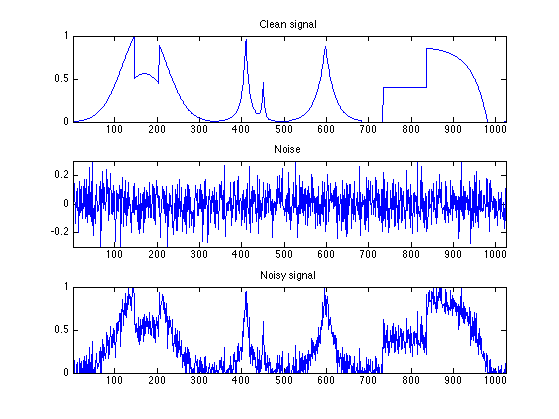Display the images.

clf;
imageplot(M0, 'Clean image', 1,3,1);
imageplot(M-M0, 'Noise', 1,3,2);
imageplot(clamp(M), 'Noisy image', 1,3,3);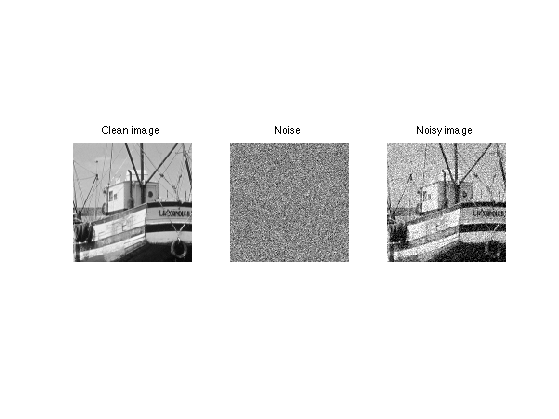Display the statistics of the noise

nbins = 51;
[h,t] = hist( M(:)-M0(:), nbins ); h = h/sum(h);
subplot(3,1,2);
bar(t,h);
axis([-sigma*5 sigma*5 0 max(h)*1.01]);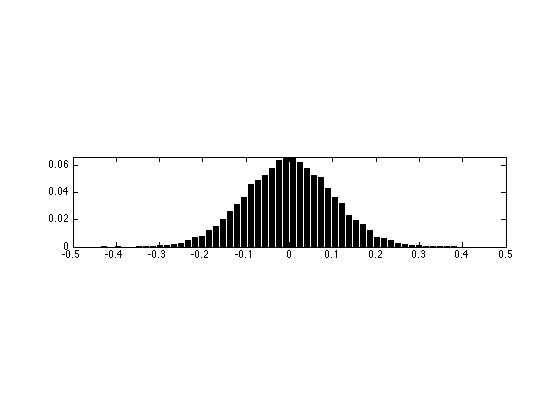A slightly different kind of noise is uniform in a given interval.

Generate noisy data with uniform noise distribution in [-a,a], with a chosen so that the variance is sigma.

a = sqrt(3)*sigma;
M = M0 + 2*(rand(N,N)-.5)*a;
f = f0 + 2*(rand(n,1)-.5)*a;


Display the signals.

clf;
subplot(3,1,1);
plot(f0); axis([1 n 0 1]);
title('Clean signal');
subplot(3,1,2);
plot(f-f0); axis([1 n -3*sigma 3*sigma]);
title('Noise');
subplot(3,1,3);
plot(f); axis([1 n 0 1]);
title('Noisy signal');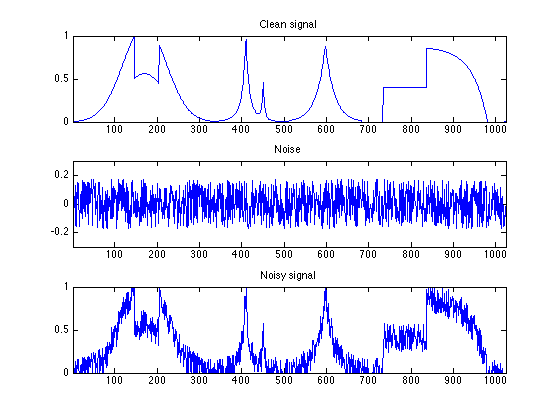Display the images.

clf;
imageplot(M0, 'Clean image', 1,3,1);
imageplot(M-M0, 'Noise', 1,3,2);
imageplot(clamp(M), 'Noisy image', 1,3,3);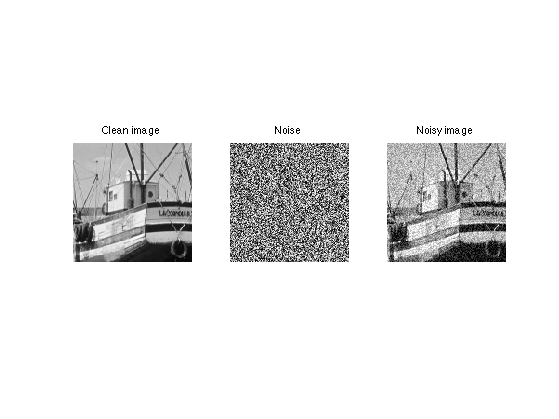Display the statistics of the noise

nbins = 51;
[h,t] = hist( M(:)-M0(:), nbins ); h = h/sum(h);
subplot(3,1,2);
bar(t,h);
axis([-sigma*5 sigma*5 0 max(h)*1.01]);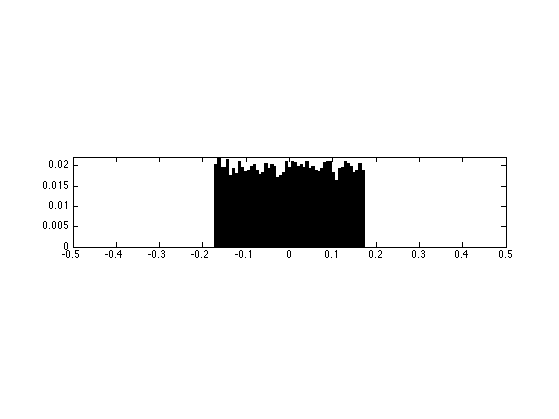## Impulse Noise

A very different noise model consist in sparse impulsions, generate by a random distribution with slowly decaying probability.

Generate noisy image with exponential distribution, with variance sigma.

W = log(rand(N,N)).*sign(randn(N,N));
W = W/std(W(:))*sigma;
M = M0 + W;


Generate noisy signal with exponential distribution, with variance sigma.

W = log(rand(n,1)).*sign(randn(n,1));
W = W/std(W(:))*sigma;
f = f0 + W;


Display the signals.

clf;
subplot(3,1,1);
plot(f0); axis([1 n 0 1]);
title('Clean signal');
subplot(3,1,2);
plot(f-f0); axis([1 n -3*sigma 3*sigma]);
title('Noise');
subplot(3,1,3);
plot(f); axis([1 n 0 1]);
title('Noisy signal');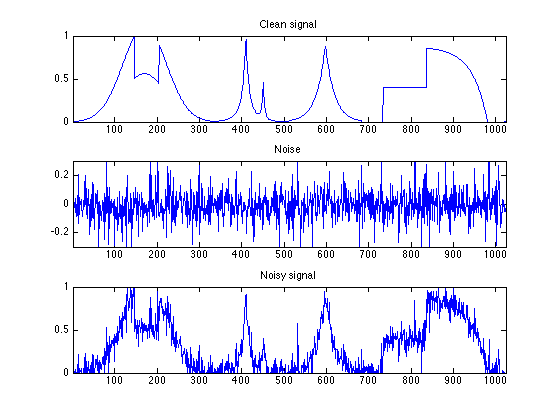Display the images.

clf;
imageplot(M0, 'Clean image', 1,3,1);
imageplot(M-M0, 'Noise', 1,3,2);
imageplot(clamp(M), 'Noisy image', 1,3,3);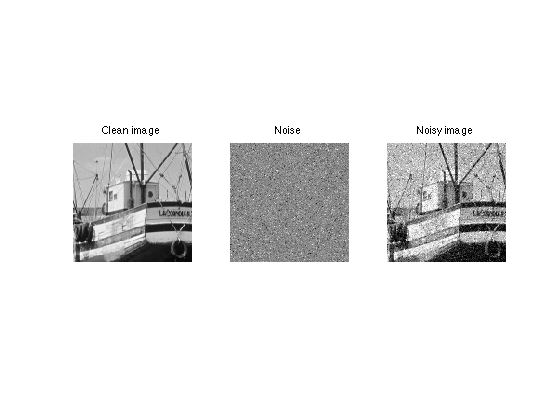Display the statistics of the noise

nbins = 51;
[h,t] = hist( M(:)-M0(:), nbins ); h = h/sum(h);
subplot(3,1,2);
bar(t,h);
axis([-sigma*5 sigma*5 0 max(h)*1.01]);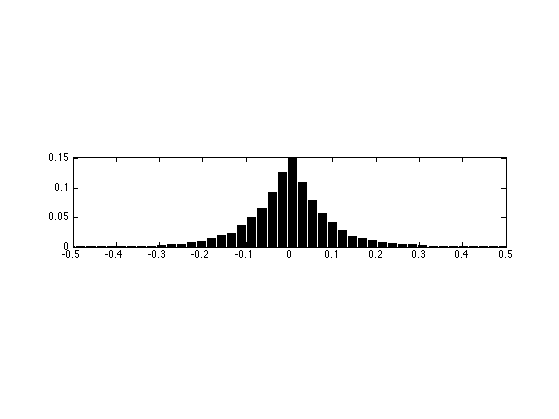## Thresholding Estimator and Sparsity

The idea of non-linear denoising is to use an orthogonal basis in which the coefficients x of the signal or image M0 is sparse (a few large coefficients). In this case, the noisy coefficients x of the noisy data M (perturbated with Gaussian noise) are x0+noise where noise is Gaussian. A thresholding set to 0 the noise coefficients that are below T. The threshold level T should be chosen judiciously to be just above the noise level.

First we generate a spiky signal.

% dimension
n = 4096;
% probability of spiking
rho = .05;
% location of the spike
x0 = rand(n,1)<rho;
% random amplitude in [-1 1]
x0 = 2 * x0 .* ( rand(n,1)-.5 );


sigma = .1;
x = x0 + randn(size(x0))*sigma;


Display.

clf;
subplot(2,1,1);
plot(x0); axis([1 n -1 1]);
set_graphic_sizes([], 20);
title('Original signal');
subplot(2,1,2);
plot(x); axis([1 n -1 1]);
set_graphic_sizes([], 20);
title('Noisy signal');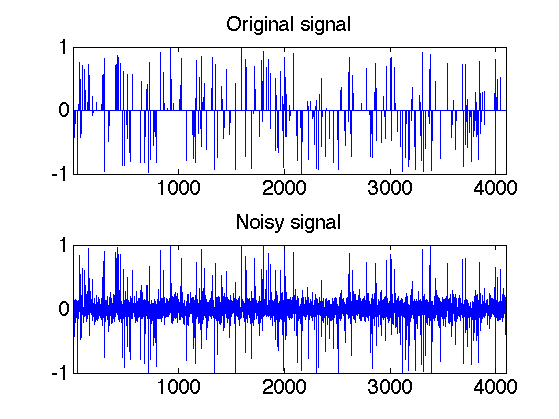Exercice 1: (check the solution) What is the optimal threshold T to remove as much as possible of noise ? Try several values of T.

exo1;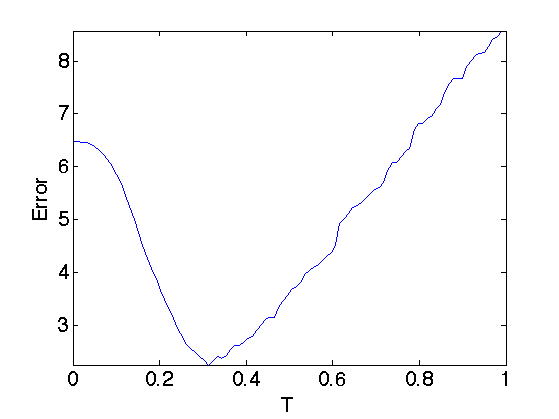In order to be optimal without knowing in advance the amplitude of the coefficients of x0, one needs to set T just above the noise level. This means that T should be roughly equal to the maximum value of a Gaussian white noise of size n.

Exercice 2: (check the solution) The theory predicts that the maximum of n Gaussian variable of variance sigma^2 is smaller than sqrt(2*log(n)) with large probability (that tends to 1 when n increases). This is also a sharp result. Check this numerically by computing with Monte Carlo sampling the maximum with n increasing (in power of 2). Check also the deviation of the maximum when you perform several trial with n fixed.

exo2;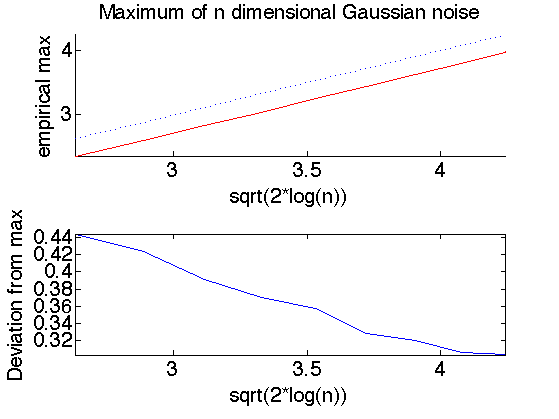## Estimating the noise level

In practice, the noise level sigma is unknown. For additive Gaussian noise, a good estimator is given by the median of the wavelet coefficients at the finer scale. An even simple estimator is given by the normalized derivate along X or Y direction

n = 256;


Generate a noisy image.

sigma = 0.06;
M = M0 + randn(n,n)*sigma;


First we extract the high frequency residual.

H = M;
H = (H(1:n-1,:) - H(2:n,:))'/sqrt(2);
H = (H(1:n-1,:) - H(2:n,:))'/sqrt(2);


Display.

clf;
imageplot(clamp(M), 'Noisy image', 1,2,1);
imageplot(H, 'Derivative image', 1,2,2);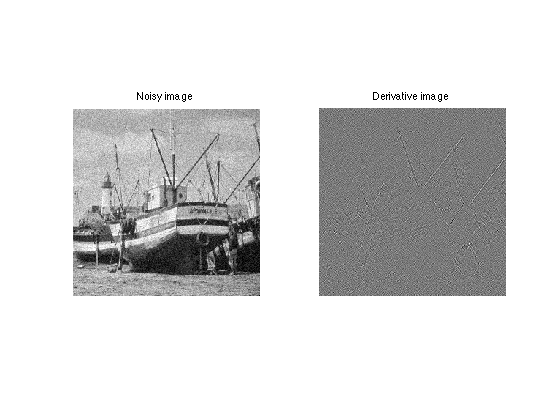Histograms.

[h,t] = hist(H(:), 100);
h = h/sum(h);


Display histogram.

clf;
bar(t, h);
axis([-.5 .5 0 max(h)]);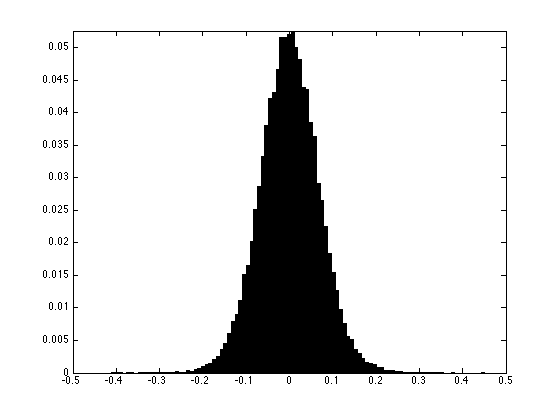The mad estimator (median of median) must be rescaled so that it gives the correct variance for gaussian noise.

sigma_est = mad(H(:),1)/0.6745;
disp( strcat(['Estimated noise level=' num2str(sigma_est), ', true=' num2str(sigma)]) );

Estimated noise level=0.066235, true=0.06